# SSAT Middle Level Math : How to add variables

## Example Questions

← Previous 1 3 4 5 6

### Example Question #1 : How To Add Variables

Maria needs exactly 47 cents.  She has 1-cent, 5-cent, 10-cent, and 25-cent coins.  What is the fewest number of coins she needs in order to make 47 cents?

Possible Answers:Correct answer:Explanation:

She needs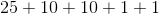to make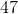cents.

### Example Question #2 : How To Add Variables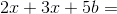Simplify this expression as much as possible

Possible Answers: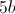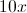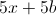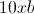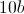Correct answer:Explanation:

You can only add like terms. Therefore, different variables are treated as different types of terms. Sinceandboth end in the variable, they can be added together. The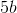cannot be added to these numbers; however, because it has a different variable. The answer is:### Example Question #1 : How To Add Variables

Simplify: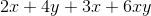Possible Answers: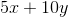The expression cannot be simplified.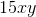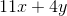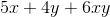Correct answer:Explanation:

The associative property of addition allows us to group the numbers with the same variables together: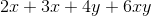The like terms in this expression are:

•and•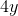•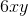Terms with different variables cannot be grouped together.

As a result, the only way to simplify this expression is to addand.

### Example Question #4 : How To Add Variables

Simplify: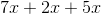Possible Answers: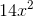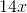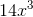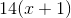Correct answer:Explanation:

When adding variables of the same type, they are simply added together, and the variable remains to the first power. This is known as combining like-terms.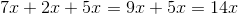Thus, the correct answer is.

### Example Question #5 : How To Add Variables

What is the sum of the fractions below?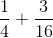Possible Answers: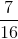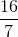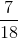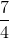Correct answer:Explanation:

In order to solve this addition problem, a common denominator must first be found.should be converted to a fraction in which the denominator is 16.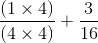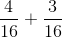Finally, add the fractions.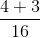### Example Question #6 : How To Add Variables

Suppose you know the values of all variables in the expression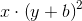and you want to evaluate the expression.

In which order will you carry out the operations?

Possible Answers:

Adding, squaring, multiplying

Squaring, adding, multiplying

Adding, multiplying, squaring

Squaring, multiplying, adding

Multiplying, adding, squaring

Correct answer:

Adding, squaring, multiplying

Explanation:

By the order of operations, the operation within parentheses, which is addition, is carried out first; of the remaining two, exponentiation - squaring here - precedes multiplication.

### Example Question #2 : How To Add Variables

Suppose you know the values of all variables in the expression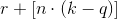and you want to evaluate the expression.

In which order will you carry out the operations?

Possible Answers:

Subtraction, addition, multiplication

Multiplication, addition, subtraction

Subtraction, multiplication, addition

Multiplication, subtraction, addition

Addition, multiplication, subtraction

Correct answer:

Subtraction, multiplication, addition

Explanation:

By the order of operations, any expressions within grouping symbols, such as parentheses and brackets, are carried out from the inside outward. Therefore, the operation in the innermost set of grouping symbols - the subtraction within parentheses - will be carried out first, followed by the remaining operation within the brackets - the multiplication. The remaining operation - the addition - is last.

### Example Question #8 : How To Add Variables

Write in base ten: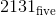Possible Answers: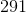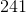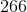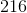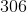Correct answer:Explanation:

In base five, each place value is a power of five, starting with 1 at the right, then, going to the left,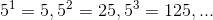.can be calculated in base ten as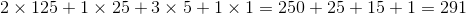.

### Example Question #9 : How To Add Variables

Add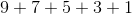in modulo 15 arithmetic.

Possible Answers:Correct answer:Explanation:

In modulo 15 arithmetic, a number is congruent to the remainder of the divison of that number by 15. Since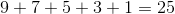and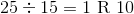,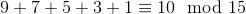.

This makes 10 the correct choice.

### Example Question #10 : How To Add Variables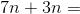Possible Answers: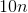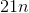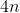Correct answer:Explanation:

Add the numbers and keep the variable: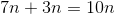Answer:← Previous 1 3 4 5 6

### All SSAT Middle Level Math Resources